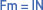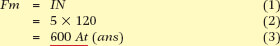# Magnetomotive Force (MMF)

Want create site? Find Free WordPress Themes and plugins.

The force required to create a magnetic field is called the magnetomotive force, abbreviated to m.m.f. with a general symbol Fm.

The magnetomotive force is the force that creates the magnetic field. The m.m.f. acts like the electromotive force in an electric circuit and is the force necessary to set up a magnetic flux in a magnetic circuit.

The m.m.f. is dependent on the current flowing in a conductor, and the magnetic field of a solenoid is dependent on the number of turns of the solenoid. The field strength of a coil is therefore proportional to the product of the current and the number of turns in the coil.

## Magnetomotive Force Unit & Formula

In pure SI units, the m.m.f. unit is the ampere, because the number of turns in a coil or solenoid is considered dimensionless. In calculations, however, the number of turns has to be included.

Generally within the electrical trades, an m.m.f. quantity will be given directly as ampere-turns (abbreviated IN), and the units will be specified as ampere-turns (At).Where:

• Fm = m.m.f. or magnetomotive force, in ampere turns
• I = current flowing in amperes
• N = number of turns in coil

Example

If a current of 5 A is flowing in a coil of 120 turns, find the value of m.m.f. creating a magnetic flux.Did you find apk for android? You can find new Free Android Games and apps.